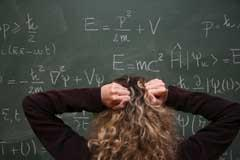David Read looks at some recent chemical education researchSource: istockphoto

The issue of maths has been troubling chemistry educators for many years. While it is clear that good mathematical skills are an essential part of any chemist's toolkit, the root causes of apparent deficiencies in the maths capabilities of chemistry students remain unclear. A study by Scott involving pupils aged 16-17 at a Scottish state school sheds light on the origins of pupils' difficulties with the mathematical aspects of chemistry.

A set of chemistry calculations were designed alongside a set of analogous maths questions, which students answered separately in chemistry and maths lessons. As the questions were testing the application of mathematical skills, students were permitted to use calculators with no time constraints applied. The questions ranged from simple one-step calculations to more complex multi-step operations, and these were administered to three mixed ability classes taught by different teachers.

The results showed there was no significant difference between students' performance on the easier questions in each set. Mistakes on these questions arose from poor understanding of basic operations such as multiplication and division, especially when applied to fractions/ratios, rather than misunderstanding of the mole concept.

As the difficulty increased, however, students' performance on the maths questions was better than on the chemistry questions. Scrutiny of individual students' answers showed that while a range of strategies were used in correct answers to chemistry questions, correct answers to the more difficult maths questions tended to be almost identical, implying the adoption of an algorithmic approach in these cases. This is indicative of poor understanding of the underlying mathematical concepts, potentially contributing to a deficiency in problem-solving abilities in other subjects. The article concludes with the suggestion that better communication between teachers in maths and science departments may alleviate the situation, with a less algorithmic approach to the teaching of maths a key part of the solution to the problem.